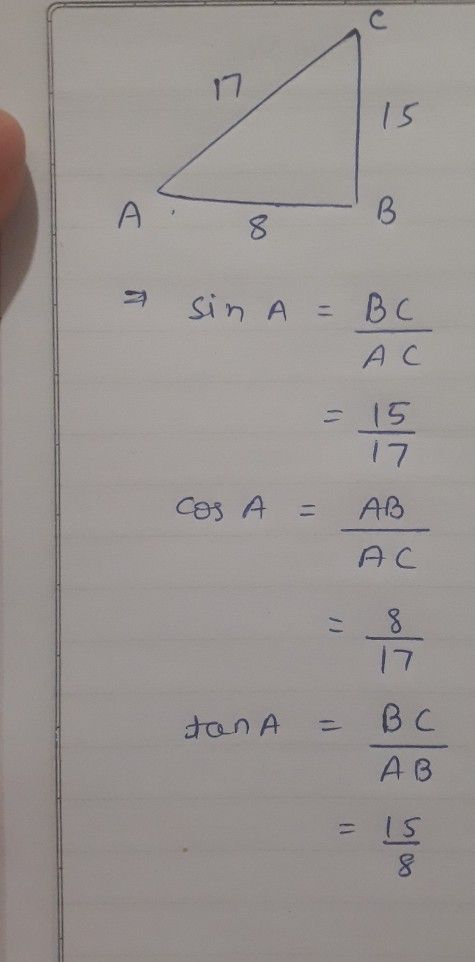Symbol
Problem(ii) $cos^{2}θ-sin^{2}θ=\left(\dfrac {21} {29}\right)$ $-\left(\dfrac {20} {29}\right)^{2}=\dfrac {41} {841}$ EXERCISE - $11.1$ In right angled triangle $ABC,8cm$ $15cm$ and $17cm$ are the lengths of AB, BC and CA respectively. Then, find sin A, cos A and tan A. The sides of a right angled triangle PQR are $PQ=7cm,$ $PR=25cm$ and $∠Q=90$ respectively. Then find, tan $nP-tanR$ In a right angled triangle $ABC$ with right angle at B, in which $a=24$ units, $b=25$ um and $∠BAC=θ$ Then, find cos 0 and $tanθ.$ If $cosA=\dfrac {12} {13}$ then find sin A and tan A $\left(A<90^{°}\right)$ If $3tanA=4,$ then find sin A and $cosA$
10th-13th grade
Trigonometry
Search count: 113
SolutionQanda teacher - Sid711Student
next solution
plzQanda teacher - Sid711
but it's against rules to ask multiple questions in a photo
I may be banned for answering
pls understandStudent
k